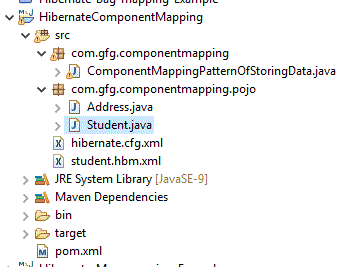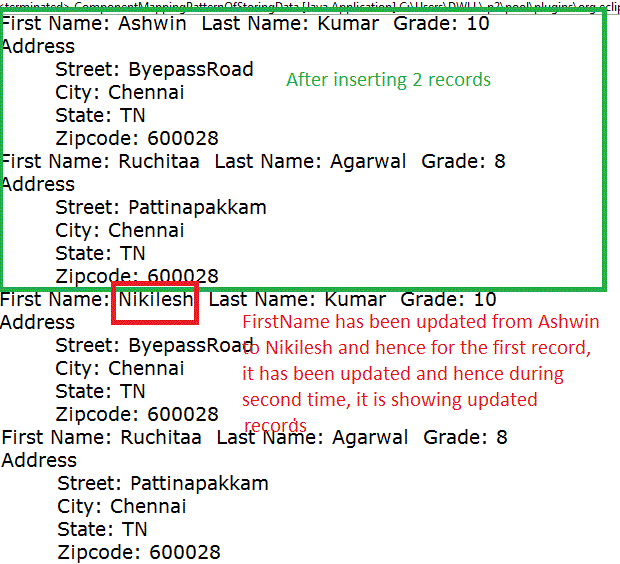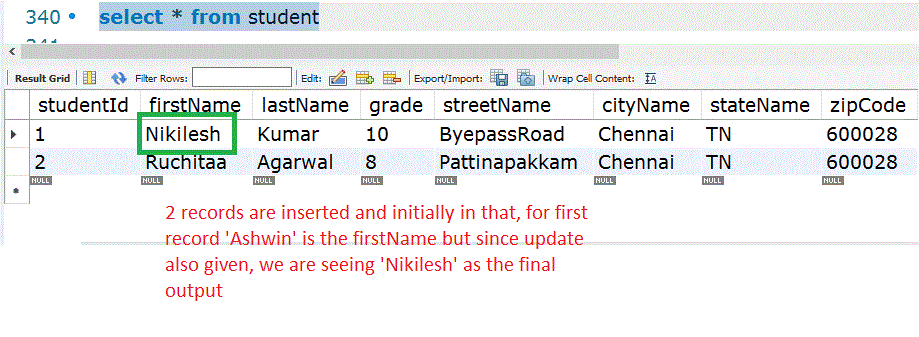Open in App
Not now

# Hibernate – Component Mapping

• Last Updated : 19 Dec, 2022

In general, a student can have an address/employee can have an address. For these kind of requirements, we can follow Component mapping. It is nothing but a class having a reference to another class as a member variable. i.e. inside the ‘student’ class, we can have the ‘address’ class as a member variable. In MySQL, only a single table is enough which holds the information of all the attributes of both classes. Here in our example, we can see ‘student’ as primary class and it has ‘address’ as member variable.

### Example Project

Project Structure:MySQL table structure for Student:

``` -- Student table
create table Student(
studentId INT NOT NULL auto_increment,
firstName VARCHAR(20) default NULL,
lastName  VARCHAR(20) default NULL,
streetName VARCHAR(40) default NULL,  -- Belongs to Address class
cityName   VARCHAR(40) default NULL, -- Belongs to Address class
stateName  VARCHAR(40) default NULL, -- Belongs to Address class
zipCode     VARCHAR(10) default NULL, -- Belongs to Address class
PRIMARY KEY (studentId)
);```

It is enough if we have only 1 table and it should have all the fields specified in the second class. Here our first class is ‘Student’ and second class is ‘Address’. Let us see the POJO classes

Student.java

## Java

 `public` `class` `Student {` `    ``// It is always good to go with each attribute of MySQL` `    ``// table` `    ``private` `int` `studentId;` `    ``private` `String firstName;` `    ``private` `String lastName;` `    ``private` `int` `grade;` `    ``// Attributes that are available in 'Address' class. By` `    ``// referring here, we can access them. As Component` `    ``// Mapping is used, it is possible` `    ``private` `Address address;` `    ``// As address itself is set as an attribute in student` `    ``// class,` `    ``// all the address related arguments can be passed` `    ``// easily via set method` `    ``public` `Address getAddress() { ``return` `address; }`   `    ``public` `void` `setAddress(Address address)` `    ``{` `        ``this``.address = address;` `    ``}`   `    ``public` `int` `getStudentId() { ``return` `studentId; }`   `    ``public` `void` `setStudentId(``int` `studentId)` `    ``{` `        ``this``.studentId = studentId;` `    ``}`   `    ``public` `String getFirstName() { ``return` `firstName; }`   `    ``public` `void` `setFirstName(String firstName)` `    ``{` `        ``this``.firstName = firstName;` `    ``}`   `    ``public` `String getLastName() { ``return` `lastName; }`   `    ``public` `void` `setLastName(String lastName)` `    ``{` `        ``this``.lastName = lastName;` `    ``}`   `    ``public` `int` `getGrade() { ``return` `grade; }`   `    ``public` `void` `setGrade(``int` `grade) { ``this``.grade = grade; }`   `    ``public` `Student(String firstName, String lastName,` `                   ``int` `grade, Address address)` `    ``{` `        ``this``.firstName = firstName;` `        ``this``.lastName = lastName;` `        ``this``.grade = grade;` `        ``this``.address = address;` `    ``}`   `    ``public` `Student() {}` `}`

## Java

 `public` `class` `Address ``extends` `Student {` `    ``private` `String streetName;` `    ``private` `String cityName;` `    ``private` `String stateName;` `    ``private` `String zipCode;` `    ``public` `Address() {}` `    ``// We should have this constructor so that address` `    ``// parameters` `    ``// can be set easily and can be accessed in student` `    ``// class` `    ``public` `Address(String street, String city, String state,` `                   ``String zipcode)` `    ``{` `        ``this``.streetName = street;` `        ``this``.cityName = city;` `        ``this``.stateName = state;` `        ``this``.zipCode = zipcode;` `    ``}` `    ``public` `String getStreetName() { ``return` `streetName; }`   `    ``public` `void` `setStreetName(String streetName)` `    ``{` `        ``this``.streetName = streetName;` `    ``}`   `    ``public` `String getCityName() { ``return` `cityName; }`   `    ``public` `void` `setCityName(String cityName)` `    ``{` `        ``this``.cityName = cityName;` `    ``}`   `    ``public` `String getStateName() { ``return` `stateName; }`   `    ``public` `void` `setStateName(String stateName)` `    ``{` `        ``this``.stateName = stateName;` `    ``}`   `    ``public` `String getZipCode() { ``return` `zipCode; }`   `    ``public` `void` `setZipCode(String zipCode)` `    ``{` `        ``this``.zipCode = zipCode;` `    ``}` `}`

This project is a maven driven project. Hence let us start with pom.xml

pom.xml

## XML

 `<``project` `xmlns``=``"http://maven.apache.org/POM/4.0.0"` `         ``xmlns:xsi``=``"http://www.w3.org/2001/XMLSchema-instance"` `         ``xsi:schemaLocation="http://maven.apache.org/POM/4.0.0 ` `                             ``https://maven.apache.org/xsd/maven-4.0.0.xsd">` `  ``<``modelVersion``>4.0.0` `  ``<``groupId``>HibernateComponentMapping` `  ``<``artifactId``>HibernateComponentMapping` `  ``<``version``>0.0.1-SNAPSHOT` `  ``<``build``>` `    ``<``sourceDirectory``>src` `    ``<``resources``>` `      ``<``resource``>` `        ``<``directory``>src` `        ``<``excludes``>` `          ``<``exclude``>**/*.java` `        ``` `      ``` `    ``` `    ``<``plugins``>` `      ``<``plugin``>` `        ``<``artifactId``>maven-compiler-plugin` `        ``<``version``>3.8.1` `        ``<``configuration``>` `          ``<``release``>9` `        ``` `      ``` `    ``` `  ``` `  ``<``dependencies``>` `        ``<``dependency``>` `            ``<``groupId``>org.hibernate` `            ``<``artifactId``>hibernate-core` `            ``<``version``>5.4.15.Final` `        ``` `        ``` `        ``<``dependency``>` `            ``<``groupId``>mysql` `            ``<``artifactId``>mysql-connector-java` `            ``<``version``>5.1.34` `        ``` `    ``` `    ``<``properties``>` `        ``<``maven.compiler.source``>1.8` `        ``<``maven.compiler.target``>1.8` `    ``` ``

For hibernate, hibernate.cfg.xml is the main configuration file

hibernate.cfg.xml

## XML

 `  ` `` `<``hibernate-configuration``>` `    ``<``session-factory``>` `    `  `          ``` `        ``<``property` `name``=``"hbm2ddl.auto"``>update  ` `        ``<``property` `name``=``"connection.driver_class"``>com.mysql.jdbc.Driver` `        ``<``property` `name``=``"connection.url"``>jdbc:mysql://localhost:3306/geeksforgeeks?serverTimezone=UTC        ` `        ``<``property` `name``=``"connection.username"``>root` `        ``<``property` `name``=``"connection.password"``>admin` `        `  `          ``` `        ``<``mapping` `resource``=``"student.hbm.xml"` `/> ` `      `  `    ``` ``

Our mapping resource is student.hbm.xml.

student.hbm.xml

## XML

 `  ` `  ` `  `  `<``hibernate-mapping``>  ` `    `  `      ``` `    ``<``class` `name``=``"com.gfg.componentmapping.pojo.Student"` `table``=``"Student"``>  `   `      ``` `    ``<``meta` `attribute` `= ``"class-description"``>` `         ``This class contains the student detail. ` `      ``   ` `  `  `      ```   `      ``<``id` `name` `= ``"studentId"` `type` `= ``"int"` `column` `= ``"studentId"``>` `         ``<``generator` `class``=``"native"``/>` `      ``    ` `  `  `      ``` `      ``<``component` `name` `= ``"address"` `class``=``"com.gfg.componentmapping.pojo.Address"``>` `         ``<``property` `name` `= ``"streetName"` `column` `= ``"streetName"` `type` `= ``"string"``/>` `         ``<``property` `name` `= ``"cityName"` `column` `= ``"cityName"` `type` `= ``"string"``/>` `         ``<``property` `name` `= ``"stateName"` `column` `= ``"stateName"` `type` `= ``"string"``/>` `         ``<``property` `name` `= ``"zipCode"` `column` `= ``"zipCode"` `type` `= ``"string"``/>` `      ``      ` `      ``<``property` `name` `= ``"firstName"` `column` `= ``"firstName"` `type` `= ``"string"``/>` `      ``<``property` `name` `= ``"lastName"` `column` `= ``"lastName"` `type` `= ``"string"``/>` `      ``<``property` `name` `= ``"grade"` `column` `= ``"grade"` `type` `= ``"int"``/>` `      `  `   ``` `            `  ``

Now let us see the main class where we can do a normal CRUD operation. Let us see the steps of addition and updation of a student in this example.

ComponentMappingPatternOfStoringData.java

## Java

 `import` `com.gfg.componentmapping.pojo.Address;` `import` `com.gfg.componentmapping.pojo.Student;` `import` `java.util.Iterator;` `import` `java.util.List;` `import` `org.hibernate.HibernateException;` `import` `org.hibernate.Session;` `import` `org.hibernate.SessionFactory;` `import` `org.hibernate.Transaction;` `import` `org.hibernate.cfg.Configuration;`   `public` `class` `ComponentMappingPatternOfStoringData {` `    ``private` `static` `SessionFactory factory;` `    ``public` `static` `void` `main(String[] args)` `    ``{`   `        ``try` `{` `            ``factory = ``new` `Configuration()` `                          ``.configure()` `                          ``.buildSessionFactory();` `        ``}` `        ``catch` `(Throwable ex) {` `            ``System.err.println(` `                ``"Failed to create sessionFactory object."` `                ``+ ex);` `            ``throw` `new` `ExceptionInInitializerError(ex);` `        ``}`   `        ``ComponentMappingPatternOfStoringData` `            ``componentMappingExample` `            ``= ``new` `ComponentMappingPatternOfStoringData();`   `        ``// Address1 ` `        ``Address address1` `            ``= componentMappingExample.addAddress(` `                ``"ByepassRoad"``, ``"Chennai"``, ``"TN"``, ``"600028"``);`   `        ``// Add student records in the database` `        ``Integer studentId` `            ``= componentMappingExample.addStudent(` `                ``"Ashwin"``, ``"Kumar"``, ``10``, address1);`   `        ``// Address2` `        ``Address address2` `            ``= componentMappingExample.addAddress(` `                ``"Pattinapakkam"``, ``"Chennai"``, ``"TN"``, ``"600028"``);`   `        ``// Add another student record in the database` `        ``Integer student2` `            ``= componentMappingExample.addStudent(` `                ``"Ruchitaa"``, ``"Agarwal"``, ``8``, address2);`   `        ``// List down all the students` `        ``componentMappingExample.listStudents();`   `        ``// Update student's name as a sample` `        ``componentMappingExample.updateStudent(studentId,` `                                              ``"Nikilesh"``);`   `        ``// List down all the students. We can see the` `        ``// updated value for the first student` `        ``componentMappingExample.listStudents();` `    ``}`   `    ``// Method to add an address record in the database` `    ``public` `Address addAddress(String street, String city,` `                              ``String state, String zipcode)` `    ``{` `        ``Session session = factory.openSession();` `        ``Transaction tx = ``null``;` `        ``Integer addressID = ``null``;` `        ``Address address = ``null``;`   `        ``try` `{` `            ``tx = session.beginTransaction();` `            ``address` `                ``= ``new` `Address(street, city, state, zipcode);` `            ``addressID = (Integer)session.save(address);` `            ``tx.commit();` `        ``}` `        ``catch` `(HibernateException e) {` `            ``if` `(tx != ``null``)` `                ``tx.rollback();` `            ``e.printStackTrace();` `        ``}` `        ``finally` `{` `            ``session.close();` `        ``}` `        ``return` `address;` `    ``}`   `    ``// Method to add a student record in the database` `    ``public` `Integer addStudent(String fname, String lname,` `                              ``int` `salary, Address address)` `    ``{` `        ``Session session = factory.openSession();` `        ``Transaction tx = ``null``;` `        ``Integer studentID = ``null``;`   `        ``try` `{` `            ``tx = session.beginTransaction();` `            ``// By using Student constructor, we can see the` `            ``// fields` `            ``Student student = ``new` `Student(fname, lname,` `                                          ``salary, address);` `            ``studentID = (Integer)session.save(student);` `            ``tx.commit();` `        ``}` `        ``catch` `(HibernateException e) {` `            ``if` `(tx != ``null``)` `                ``tx.rollback();` `            ``e.printStackTrace();` `        ``}` `        ``finally` `{` `            ``session.close();` `        ``}` `        ``return` `employeeID;` `    ``}`   `    ``// Method to list all the student detail` `    ``public` `void` `listStudents()` `    ``{` `        ``Session session = factory.openSession();` `        ``Transaction tx = ``null``;`   `        ``try` `{` `            ``tx = session.beginTransaction();` `            ``// Take the data from Student table` `            ``List students` `                ``= session.createQuery(``"FROM Student"``)` `                      ``.list();` `            ``// Iterate the details and display them` `            ``for` `(Iterator iterator = students.iterator();` `                 ``iterator.hasNext();) {` `                ``Student student = (Student)iterator.next();` `                ``System.out.print(``"First Name: "` `                                 ``+ student.getFirstName());` `                ``System.out.print(``"  Last Name: "` `                                 ``+ student.getLastName());` `                ``System.out.println(``"  Grade: "` `                                   ``+ student.getGrade());` `                ``Address add = student.getAddress();` `                ``System.out.println(``"Address "``);` `                ``System.out.println(``"\tStreet: "` `                                   ``+ add.getStreetName());` `                ``System.out.println(``"\tCity: "` `                                   ``+ add.getCityName());` `                ``System.out.println(``"\tState: "` `                                   ``+ add.getStateName());` `                ``System.out.println(``"\tZipcode: "` `                                   ``+ add.getZipCode());` `            ``}` `            ``tx.commit();` `        ``}` `        ``catch` `(HibernateException e) {` `            ``if` `(tx != ``null``)` `                ``tx.rollback();` `            ``e.printStackTrace();` `        ``}` `        ``finally` `{` `            ``session.close();` `        ``}` `    ``}`   `    ``// Method to update name for a given student` `    ``public` `void` `updateStudent(Integer studentId,` `                              ``String firstName)` `    ``{` `        ``Session session = factory.openSession();` `        ``Transaction tx = ``null``;`   `        ``try` `{` `            ``tx = session.beginTransaction();` `            ``Student student = (Student)session.get(` `                ``Student.``class``, studentId);` `            ``student.setFirstName(firstName);` `            ``session.update(student);` `            ``tx.commit();` `        ``}` `        ``catch` `(HibernateException e) {` `            ``if` `(tx != ``null``)` `                ``tx.rollback();` `            ``e.printStackTrace();` `        ``}` `        ``finally` `{` `            ``session.close();` `        ``}` `    ``}` `}`

On running the project, we can see the below output.

Output:MySQL output also matches the same### Conclusion

By using component mapping, the association of class elements can be done easily and in MySQL, it is enough if we have a single table only to satisfy the component mapping.

My Personal Notes arrow_drop_up
Related Articles